1604127900

# Reverse Engineering Graph Convolutional Networks

This blog post will summarise the paper “ Simplifying Graph Convolutional Networks ”, which tries to reverse engineer the Graph Convolutional Networks. So, let us evolve Graph Convolutional Networks backward.

Graphs are pervasive models of structures. They are everywhere, from social networks to the chemistry molecule. Various things can be represented in terms of graphs. However, applying Machine learning to these structures is something that didn’t come directly to us. Everything in Machine learning came from a small simple idea or model which was made complex with time as per the need. Just as an example, initially, we had Perceptron which evolved to Multi-Layer perception, similarly, we had image filters that evolved to non-linear CNNs, and so on. However, Graph Convolutional Networks, referred to as GCN, were something we derived directly from existing ideas and had a more complex start. Thus, to debunk the GCNs, the paper tries to reverse engineer the GCN and proposes a simplified linear model called Simple Graph Convolution (SGC). SGC as when applied gives comparable performance to GCNs and is faster than even the Fast-GCN.

## Basic Notations

Inputs to the Graph convolutional network are:

1. Node Labels

**Adjacency matrix: **The adjacency matrix **A **is **n x n,**matrix where n is the number of nodes, with a(i,j) = 1 if node i is connected to node j else a(i,j) = 0. If edge is weighted then a(i,j) = edge weight.

**Diagonal Matrix: **Diagonal matrix **D **is n x n matrix with d(i,i) = sum of ith row of adjacency matrix.

**Input features: **X is an input feature matrix of size n x c with c as the number of classes.

Let us see how GCNs actually work before reverse engineering it.

#machine-learning #graph-convolution #graph-neural-networks #gcn #neural-networks

## Buddha Community1604127900

## Reverse Engineering Graph Convolutional Networks

This blog post will summarise the paper “ Simplifying Graph Convolutional Networks ”, which tries to reverse engineer the Graph Convolutional Networks. So, let us evolve Graph Convolutional Networks backward.

Graphs are pervasive models of structures. They are everywhere, from social networks to the chemistry molecule. Various things can be represented in terms of graphs. However, applying Machine learning to these structures is something that didn’t come directly to us. Everything in Machine learning came from a small simple idea or model which was made complex with time as per the need. Just as an example, initially, we had Perceptron which evolved to Multi-Layer perception, similarly, we had image filters that evolved to non-linear CNNs, and so on. However, Graph Convolutional Networks, referred to as GCN, were something we derived directly from existing ideas and had a more complex start. Thus, to debunk the GCNs, the paper tries to reverse engineer the GCN and proposes a simplified linear model called Simple Graph Convolution (SGC). SGC as when applied gives comparable performance to GCNs and is faster than even the Fast-GCN.

## Basic Notations

Inputs to the Graph convolutional network are:

1. Node Labels

**Adjacency matrix: **The adjacency matrix **A **is **n x n,**matrix where n is the number of nodes, with a(i,j) = 1 if node i is connected to node j else a(i,j) = 0. If edge is weighted then a(i,j) = edge weight.

**Diagonal Matrix: **Diagonal matrix **D **is n x n matrix with d(i,i) = sum of ith row of adjacency matrix.

**Input features: **X is an input feature matrix of size n x c with c as the number of classes.

Let us see how GCNs actually work before reverse engineering it.

#machine-learning #graph-convolution #graph-neural-networks #gcn #neural-networks1595691960

## How Graph Convolutional Networks (GCN) Work

In this post, we’re gonna take a close look at one of the well-known Graph neural networks named GCN. First, we’ll get the intuition to see how it works, then we’ll go deeper into the maths behind it.

## Why Graphs?

Many problems are graphs in true nature. In our world, we see many data are graphs, such as molecules, social networks, and paper citations networks.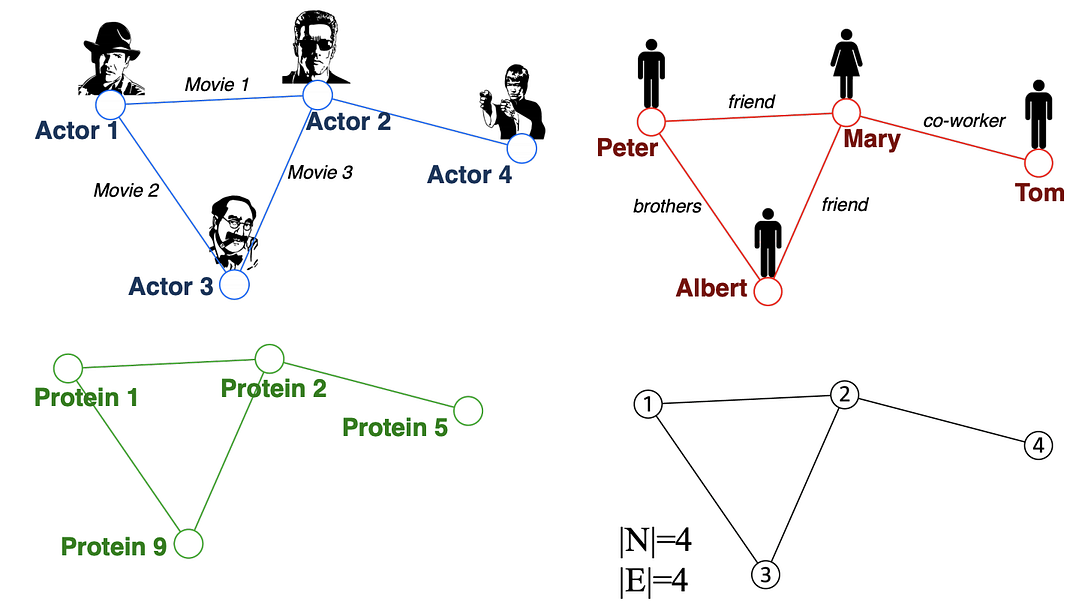Examples of graphs. (Picture from )

• Node classification: Predict a type of a given node
• Community detection: Identify densely linked clusters of nodes
• Network similarity: How similar are two (sub)networks

## Machine Learning Lifecycle

In the graph, we have node features (the data of nodes) and the structure of the graph (how nodes are connected).

For the former, we can easily get the data from each node. But when it comes to the structure, it is not trivial to extract useful information from it. For example, if 2 nodes are close to one another, should we treat them differently to other pairs? How about high and low degree nodes? In fact, each specific task can consume a lot of time and effort just for Feature Engineering, i.e., to distill the structure into our features.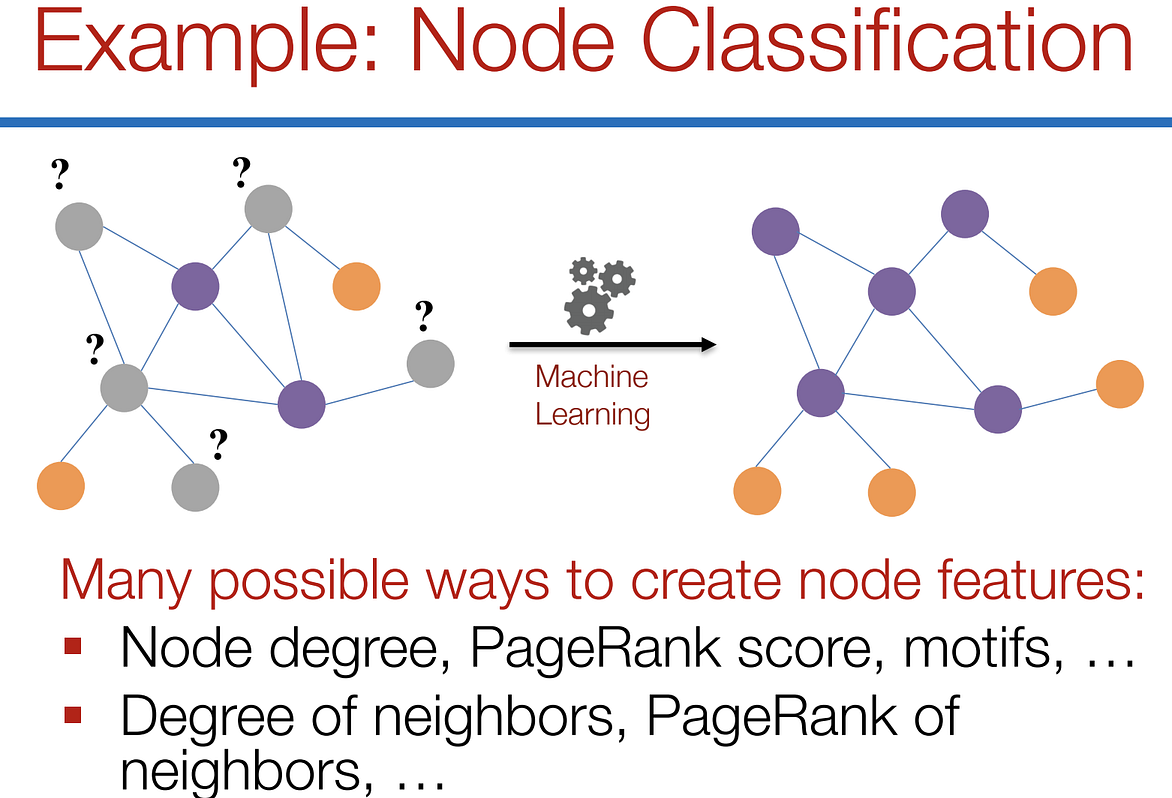Feature engineering on graphs. (Picture from )

It would be much better to somehow get both the node features and the structure as the input, and let the machine to figure out what information is useful by itself.

That’s why we need Graph Representation Learning.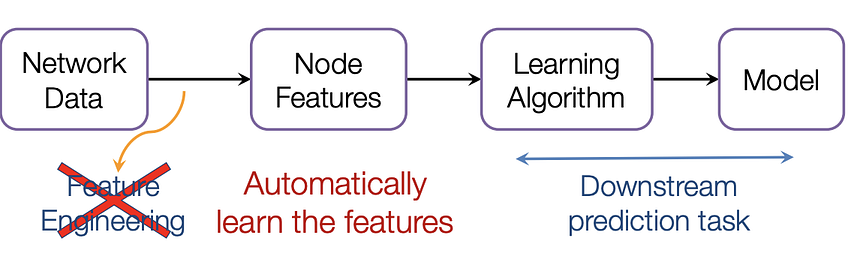We want the graph can learn the “feature engineering” by itself. (Picture from )

# Graph Convolutional Networks (GCNs)

Paper: Semi-supervised Classification with Graph Convolutional Networks(2017) 

GCN is a type of convolutional neural network that can work directly on graphs and take advantage of their structural information.

it solves the problem of classifying nodes (such as documents) in a graph (such as a citation network), where labels are only available for a small subset of nodes (semi-supervised learning).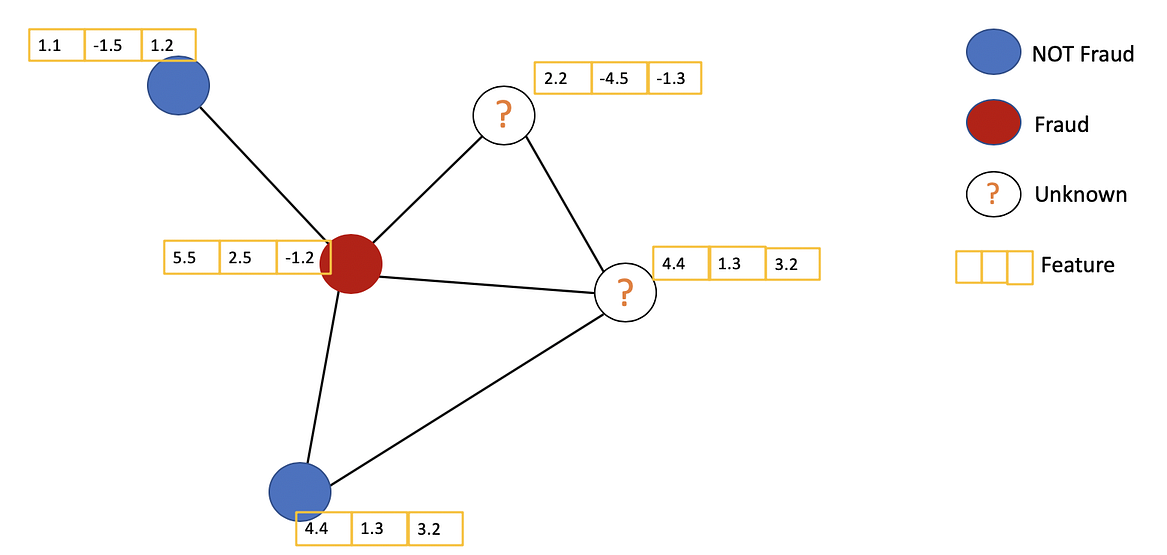Example of Semi-supervised learning on Graphs. Some nodes dont have labels (unknown nodes).

#graph-neural-networks #graph-convolution-network #deep-learning #neural-networks1602838800

## SimGNN: Similarity Computation via Graph Neural Networks

This post will summarize the paper SimGNN which aims for fast graph similarity computation. Graphs are structures that are used to link different entities that we call nodes using relationships called edges. Graphs exist everywhere from bonds between the atoms to friends on Facebook, all these scenarios can be represented as a graph. One of the fundamental graph problems includes finding similarity between graphs. The similarity between graphs can be defined using these metrics :

1. Graph Edit Distance
2. Maximum Common Subgraph

However, currently available algorithms that are used to calculate these metrics have high complexities and it is not yet possible to compute exact GED using these for graphs having more than 16 nodes.

Some ways to compute these metrics are :

1. Pruning verification Framework
2. Approximating the GED in fast and heuristic ways

SimGNN follows another approach to tackle this problem i.e turning similarity computation problem into a learning problem.

Before getting into how SimGNN works, we must know the requirements to be satisfied by this model. It includes :

1. Representation Invariant: Different representations of the same graph should give the same results.
2. **Inductive: **Should be able to predict results for unseen graphs.
3. Learnable: Must work on different similarity metrics like GED and MCS

**SimGNN Approach: **To achieve the above-stated requirements, SimGNN uses two strategies

1. Design Learnable Embedding Function: This maps the graph into an embedding vector, which provides a global summary of a graph. Here, some nodes of importance are selected and used for embedding computation. (less time complexity)
2. Pair-wise node comparison: The above embedding are too coarse, thus further compute the pairwise similarity scores between nodes from the two graphs, from which the histogram features are extracted and combined with the graph level information. (this is a time-consuming strategy)

#graph-edit-distance #machine-learning #graph-neural-networks #graph-convolution-network1602410400

## What are graph convolutional networks?

A typical feedforward neural network takes the features of each data point as input and outputs the prediction. The neural network is trained utilizing the features and the label of each data point in the training data set. Such a framework has been shown to be very effective in a variety of applications, such as face identification, handwriting recognition, object detection, where no explicit relationships exist between data points. However, in some use cases, the prediction for a data point v(i) can be determined not only by its own features but also by the features of other data points v(j) when the relationship between v(i) and v(j) is given. For example, the topic of a journal paper (e.g computer science, physics, or biology) can be inferred from the frequency of words appearing in the paper. On the other hand, the reference in a paper can also be informative when predicting the topic of the paper. In this example, not only do we know the features of each individual data point (the word frequency), we also know the relationship between the data points (citation relation). So how can we combine them to increase the accuracy of the prediction?

By applying graph convolutional networks (GCN), the features of an individual data point and its connected data points will be combined and fed into the neural network. Let’s use the paper classification problem again as an example. In a citation graph (Fig. 1), each paper is represented by a vertex in the citation graph. The edges between the vertices represent the citation relationships. For simplicity, the edges are treated as undirected. Each paper and its feature vector are denoted as v_i and x_i respectively. Following the GCN model by Kipf and Welling , we can predict the topics of papers using a neural network with one hidden layer with the following steps: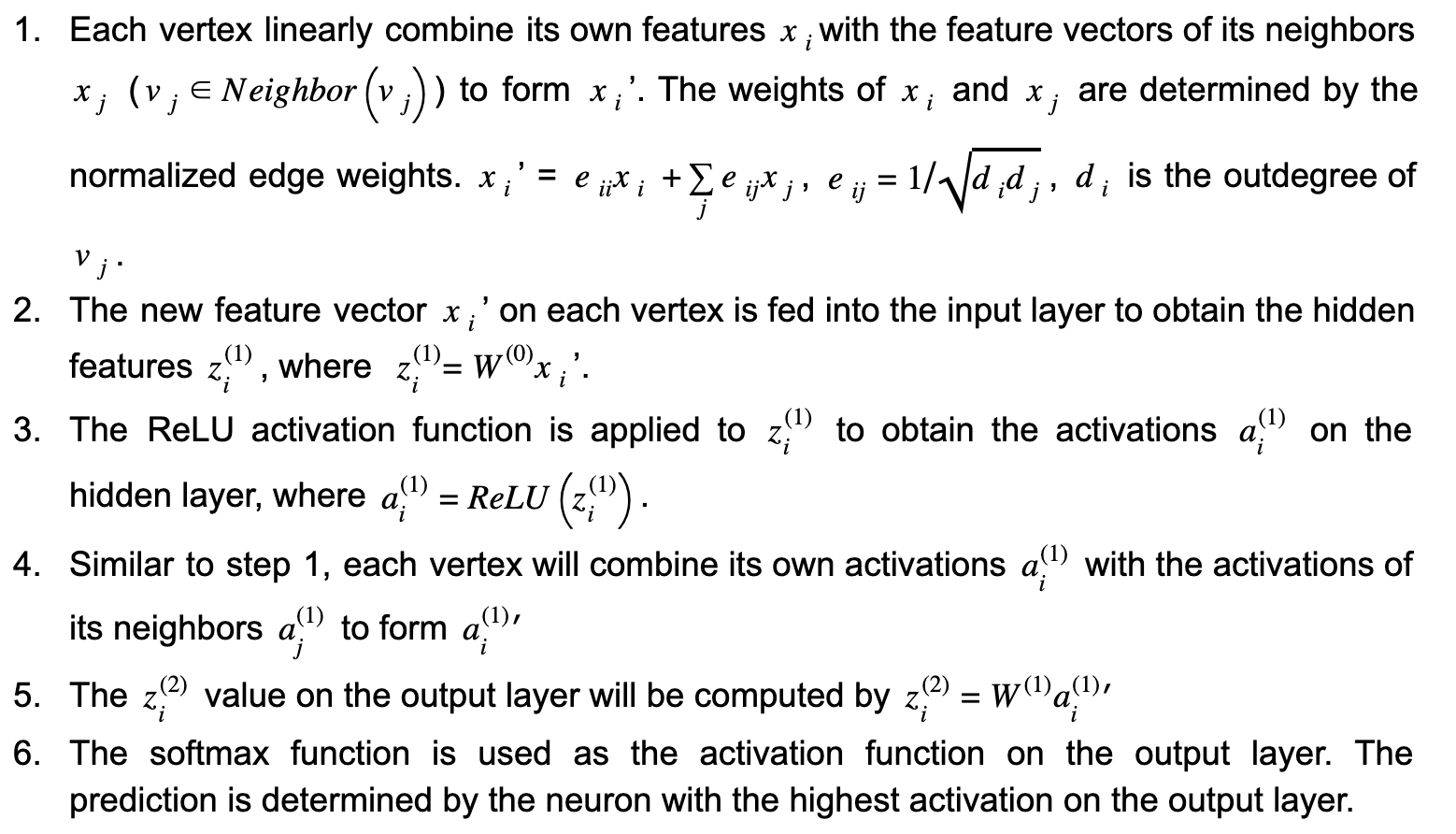Figure 1.(Image by Author) The architecture of graph convolutional networks. Each vertex vi represents a paper in the citation graph. xi is the feature vector of vi. W(0) and W(1) are the weight matrices of the 3-layer neural network. A, D, and I are the adjacency matrix, outdegree matrix, and identity matrix respectively. The horizontal and vertical propagations are highlighted in orange and blue respectively.

In the above workflow, steps 1 and 4 perform horizontal propagation where the information of each vertex is propagated to its neighbors. While steps 2 and 5 perform vertical propagation where the information on each layer is propagated to the next layer. (see Fig. 1) For a GCN with multiple hidden layers, there will be multiple iterations of horizontal and vertical propagations. It is worth noting that each time horizontal propagation is performed, the information of a vertex is propagated one-hop further on the graph. In this example, the horizontal propagation is performed twice (steps 2 and 4), so the prediction of each vertex not only depends on its own features, but also the features of all the vertices within 2-hop distance from it. Additionally, since the weight matrix W(0) and W(1)are shared by all the vertices, the size of the neural network does not have to increase with the graph size, which makes this approach scalable.

#classification #machine-learning #graph-convolution-network #semi-supervised-learning #graph-database1604086260

## The simplest explanation of Graph Convolutional Neural Networks

This blog post is a summarized version of GCNNs from the paper “From Graph Filters to Graph Neural Networks. Graphs are structures that are used to link different entities that we call nodes using relationships called edges. Graphs exist everywhere from bonds between the atoms to friends on Facebook, all these scenarios can be represented as a graph. Thus we can say that graphs are pervasive models of structure. But we are good at running CNNs, convolutional neural networks, on structured graphs like images.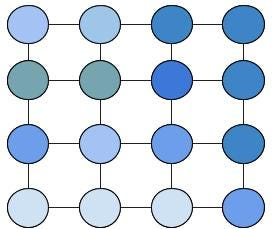Fig1. Images as a Structured graph

While actual graphs that we want to run Neural Network over, look like these.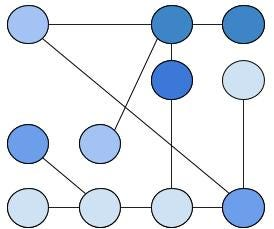Fir2. Unstructured Graph. Real ones are more complex.

Graph Neural Networks are the tool of choice for performing machine learning on graphs. So we need to generalize convolutions on graphs to create a layered Graph (convolutional) neural network.

## Convolutions on Graphs

We know the convolutions of signals in time and space (images). These two can be imagined as structured graphs, i.e a signal in time can be visualized as a single list like a graph structure while an image as shown in the image above (Fig 1). Let me define the convolution in the matrix form for a single list like the graph. It is equal to: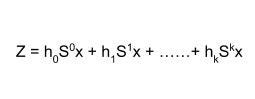Convolution output

where S¹ is a shift by 1, S² is a shift by two and so on, h(i) is the filter coefficient and x is our signal. Observe here, how the matrix convolution is just a product with the shifted signal. A similar observation is seen for images convolution (Yes it is the same as in the link given). Similarly, convolution in graphs can also be written in the same form where S is the adjacency matrix.

#graph-filters #algorithms #ai #neural-networks #graph-convolution